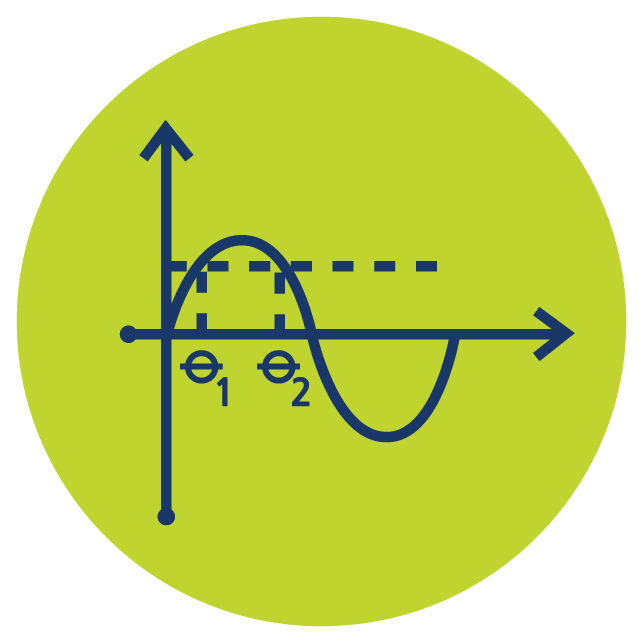Select Page# Trigonometric Equations

The Trigonometric Equations online course firstly helps you develop core trigonometry skills which are then used to solve a wide range of trigonometric equations. Students master these techniques first and consequently solve the equations themselves. View our classroom with this PREVIEW or learn more and start your FREE one week Trigonometric Equations TRIAL.

### Who is the Trigonometric Equations Online Course For?

The Trigonometric Equations online course is ideal for students who are learning trigonometric equations for the first time. However, if you have some experience you can use this course to review techniques and then practice. Trigonometric Equations can subsequently be used in a variety of disciplines and technical careers. View the CLASSROOM.

### What is Taught in the Trigonometric Equations Online Course?

Radians, Trigonometric Functions, Reciprocal Trigonometric Functions, Hyperbolic Functions, The CAST Diagram, Exact Values, Trigonometric Equations with Identities, Equations with Reciprocal Functions, Equations with Hyperbolic Functions. Master these topics with our step by step on-demand videos and practice question worksheets – basically like having your own Trig Equations tutor 24/7. View the full Trigonometric Equations course SYLLABUS.

### What Will I Learn in This Course?

You will develop a deep understanding of the trigonometric functions and related concepts and will then apply this to solve various types of trigonometric equation. Firstly, you master entry level questions and soon move onto those involving trigonometric identities and hyperbolic functions. Download FREE TRIGONOMETRY QUESTION WORKSHEETS.

### How Does Trigonometric Equations Compare to Trigonometry?

The Trigonometry online course covers many aspects of trigonometry and is certainly an ideal pre-requisite for studying Trigonometric Equations. However, the Trigonometric Equations course focuses on the trigonometry concepts required for solving equations. Learn more about TRIGONOMETRY.

### What’s Next?

Trigonometric Equations are used in many disciplines such as science, technology and engineering, and in many advanced Math courses. So, having these skills in place is a great foundation for henceforth pursuing these areas.

## Core Calculus & Beyond Course Bundle

Access all 6 courses in the bundle Core Calculus & Beyond to easily review older topics or jump ahead when ready.